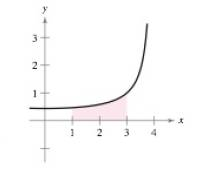Chapter 8.5, Problem 44E

Chapter
Section
Textbook Problem

# Area In Exercises 41-44, use partial fractions to find the area of the given region. y = 7 16 − x 2To determine

To calculate: The area of the provided region by using partial fractions.

Explanation

Given:

The graph of the region

Formula used:

1. [F(x)]ab=F(b)F(a)

2. [f(u)±g(u)]du=f(u)du±g(u)du

3. 1xdx=lnx+C

Calculation:

Consider the provided function.

y=716x2

For the above function area is given by, 13716x2dx.

Now,

716x2dx=71(4+x)(4x)dx

By using partial fraction method we get,

1(4+x)(4x)=A4+x+B4x1(4+x)(4x)=A(4x)+B(4+x)(4+x)(4x)

By simplifying further, we get,

1=A(4x)+B(4+x)

.At x=4,

A(4(4))+B(4+(4))=18A=1A=18

At x=4,

A(44)+B(4+4)=18B=1B=18

So, by substituting the value of A and B we get,

116x2=18(4+x)+18(4x)=18(4+x)+18(4x)........

### Still sussing out bartleby?

Check out a sample textbook solution.

See a sample solution

#### The Solution to Your Study Problems

Bartleby provides explanations to thousands of textbook problems written by our experts, many with advanced degrees!

Get Started

#### Find the value of the sum. 28. i=2423i

Single Variable Calculus: Early Transcendentals, Volume I

#### let f(x) = x3 + 5, g(x) = x2 2, and h(x)= 2x + 4. Find the rule for each function. 5. fg

Applied Calculus for the Managerial, Life, and Social Sciences: A Brief Approach

#### In problems 1-16, solve each equation. 14.

Mathematical Applications for the Management, Life, and Social Sciences

#### In Exercises 1-22, evaluate the given expression. P(5,3)

Finite Mathematics for the Managerial, Life, and Social Sciences